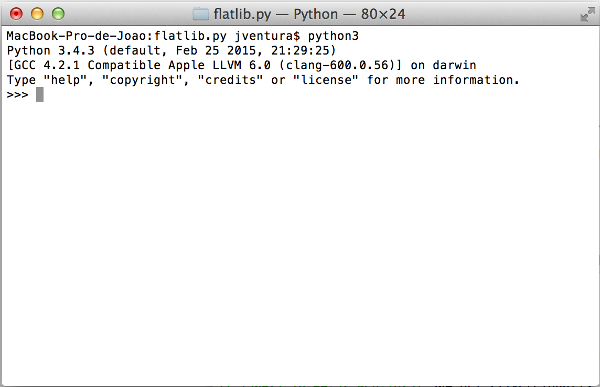# Introduction to Python¶

The purpose of this tutorial is to show you how you can quickly start working with the Python programming language. It assumes that you have already succesfully installed Python in your computer. If you already know how to use Python, you can skip this tutorial.

## Interactive interpreter¶

Python is a general purpose programming language that bundles an interactive interpreter. To start the interactive interpreter, open the terminal (command prompt in Windows) and execute `python3` (or `py` in Windows)In the interactive interpreter, you can enter commands and the Python interpreter will execute those commands and return the answers.

## Variables¶

In computer languages, variables are reserved memory locations to store values. Basically, when you create a variable, the Python interpreter allocates memory and stores a value in that memory location. The equal sign (=) is used to assign values to variables. Try the following in your interpreter:

```>>> number = 100
>>> miles = 1000.0
>>> name = "John"
>>> print(number)
100
>>> print(miles)
1000.0
>>> print(name)
John
```

You can assign integer numbers to variables, floating point numbers and strings.

## Basic Operations¶

Since variables may include numbers, strings or other objects, Python allows us to do some basic operations on variables, such as adding, subtracting, multiplying or divinding. Some of those operations make more sense on numbers, but others may be applied in strings as well.

Try the following operations on numbers:

```>>> num1 = 10
>>> num2 = 20
>>> num1 + num2
30
>>> num1 - num2
-10
>>> num1 * num2
200
>>> num1 / num2
0.5
```

Now let’s see how it works for strings:

```>>> name1 = 'Homer'
>>> name2 = 'Simpson'
>>> name1 + name2
HomerSimpson
>>> name1 - name2
Traceback (most recent call last):
File "<stdin>", line 1, in <module>
TypeError: unsupported operand type(s) for -: 'str' and 'str'
```

Some operations do not make sense for strings.

## Assignment operator¶

Something very important in programming languages is the assigment operator. We have already discussed it above when we assigned numbers and strings to variables using the equal operator (=). The important thing to notice is that we can assign the result of an operator to a variable like in the following example:

```>>> num1 = 10
>>> num2 = 20
>>> result = num1 + num2
>>> print(result)
30
>>> result   # This also works in the interpreter
30
```

## Python lists and dictionaries¶

In Python, there are two special data structures called lists and dictionaries. A list is basically a sequence of variables which can be or not of the same data type. Here is a simple example:

```>>> mylist = [-5, 0.3, 2.5, 33]
>>> mylist
-5
>>> mylist
0.3
>>> mylist + mylist
-4.7
```

We can access the individual contents of a list by referring to the index number between brackets. For instance, `mylist` returns the contents of mylist at index 1. Lists are zero-based.

A dictionary is a data structure somewhat similar to lists but which do not represent sequences of variables. Here is an example for dictionaries:

```>>> mydict = {'name': 'John Doe', 'age': 32, 'gender': 'male'}
>>> mydict['name']
John Doe
>>> mydict['age']
32
>>> mydict['age'] = 32 * 2
>>> mydict['age']
64
```

Similarly to lists, you can access the individual contents of a dictionary using the index value between brackets. But unlike lists, you can also use strings or other objects as key.

## Python modules¶

Python provides different functionalities organized by modules. A module is a file containing Python definitions, statements and functions.

Python comes with a library of standard modules which provides many functionalities. To access a module you must explicitly import it using the import command. Here is an example of importing the math module to use some of its functions:

```>>> import math
>>> math.factorial(10)
3628800
>>> math.log(20)
2.995732273553991
```

The Python Library Reference describes the standard library that is distributed with Python, and can be found at https://docs.python.org/3.4/library/index.html.